Courses

# Test: Refrigeration Cycles & Systems - 3

## 30 Questions MCQ Test Thermodynamics | Test: Refrigeration Cycles & Systems - 3

Description
This mock test of Test: Refrigeration Cycles & Systems - 3 for Mechanical Engineering helps you for every Mechanical Engineering entrance exam. This contains 30 Multiple Choice Questions for Mechanical Engineering Test: Refrigeration Cycles & Systems - 3 (mcq) to study with solutions a complete question bank. The solved questions answers in this Test: Refrigeration Cycles & Systems - 3 quiz give you a good mix of easy questions and tough questions. Mechanical Engineering students definitely take this Test: Refrigeration Cycles & Systems - 3 exercise for a better result in the exam. You can find other Test: Refrigeration Cycles & Systems - 3 extra questions, long questions & short questions for Mechanical Engineering on EduRev as well by searching above.
QUESTION: 1

### The flash chamber in a single stage simple vapour compression cycle serves to

Solution:

Mass flow of refrigerant through the evaporator gets reduced.

QUESTION: 2

Solution:
QUESTION: 3

### In a domestic refrigerator, periodic defrosting is required because frosting

Solution:
QUESTION: 4

Pressure drop due to ‘wire drawing' in actual vapour compression cycle is observed at

Solution:
QUESTION: 5

Large sized industrial air-conditioning plant are

Solution:

Large sized industrial air conditioning system are generally water cooled

QUESTION: 6

The COP of a Carnot refrigeration cycle decreases on

Solution:

It can be noted that the maximum possible Cofficient of Performance in any application can be obtained when:
(i) The cold body Temperature (Tc) should be as high as possible and
(ii) The hot body Temperature (Th) should be as low as possible.
Hence most effective way to increase the COP is by increasing the temperature of cold body and at the some time decreasing the temperature of hot body.
If opposite is done i.e., decreasing the temperature of cold body and increasing the temperature of hot body, COP of a Carnot refrigeration cycle decreases drastically,

QUESTION: 7

COP of a domestic refrigerator in comparison to domestic air conditioner will be

Solution:

Due to low temperature in domestic refrigerator COP of domestic refrigerator < COP of domestic air-conditioner.

QUESTION: 8

The correct sequence of Vapour Compression (VC), Vapour Absorption (VA) and Steam Injection (SE) refrigeration cycles in increasing order of the COP is

Solution:
QUESTION: 9

In steam-injection system compression is achieved by

Solution:
QUESTION: 10

Which one of the following sequence is correct in vapour compression cycle​

Solution: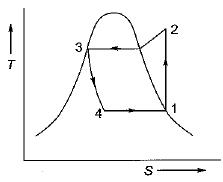Hence sequence followed is
1 - 2 : Isentropic compression (in compressor)
2 - 3 : Constant pressure heat rejection (in condenser)
3 - 4 : lso - enthalpic expansion (in capillary tube)
4 - 1 : Constant pressure heat absorption (in evaporator)

QUESTION: 11

In a vapour-compression refrigeration cycle, if h1 and h2 denote the enthalpies at inlet and exit of the compressor respectively, h3 is the enthalpy at the exit of the condenser and h4 is the enthalpy at the inlet of the evaporator, then COP for the cycle is

Solution: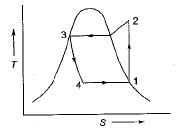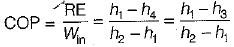(Since h4 = h3)

QUESTION: 12

Formation of frost on evaporator of refrigerator

Solution:

Frost decreases the cooling efficiency due to poor heat transfer rate.

QUESTION: 13

In a vapour compression cycle, the refrigerant immediately after expansion valve is

Solution: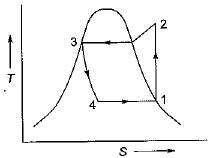At point 4 i.e. refrigerant immediately after expansion valve is wet vapour.

QUESTION: 14

Sub-cooling in a refrigeration cycle have effect of

Solution:

Effect of Sub-cooling:
1. Increase in refrigeration effect
2. Horse power per ton is reduced
3. Increase in COP

QUESTION: 15

In a vapour compression refrigeration system, the effect of superheating the vapour before suction to the compressor

Solution:
QUESTION: 16

Superheating in refrigeration cycle

Solution:

Effect of Superheating:
1. Work input increases
2. Increase in refrigeration effect

QUESTION: 17

Solid CO2 is produced by

Solution:

There are two method of manufacturing of solid CO2
1. Simple vapour compression cycle
2. Pressure snow chamber method
Power requirement for the simple vapour compression cycle is of the order of 400-500 J-hr per ton of solid CO2; one of the main reason for such high power consumption is the high pressure ratio that is nearly equal to 70.
Another problem in the process of manufacture is the blocking of expansion device by the formation of dry ice due to these difficulties, snow chamber method is most commonly used.

QUESTION: 18

Flash chamber is used in refrigeration for which one of the following?

Solution:
QUESTION: 19

The thermodynamic cycle used in aircraft refrigeration is

Solution:

In aircraft refrigeration system Beil Coleman cycle is used. It is a reversed Brayton Cycle.

QUESTION: 20

In aircraft, air refrigeration cycle is used because of

Solution:

In aircraft, air refrigeration cycle is used due to low weight per tonne of refrigeration on the other hand COP of this cycle is very low.

QUESTION: 21

Bell Coleman cycle consists of

Solution: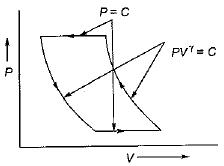Hence Bell Coleman cycle consists of two reversible isentropic process and two reversible isobaric process.

QUESTION: 22

In an aircraft refrigeration system, pressure at the cooling turbine outlet is equal to

Solution:
QUESTION: 23

Which of the following machines can be used to obtain refrigeration at the place where there is no electric power?

Solution:

Vapour absorption refrigeration is heat operated refrigeration system and can operate by taking heat from the solar system.

QUESTION: 24

In the absorption refrigeration cycle, the compressor of the vapour compression refrigeration cycle is replaced by

Solution:

In the absorption refrigeration system the compressor of the vapour compression refrigeration cycle is replaced by pump, absorber and generator.

QUESTION: 25

An electrolux refrigerator uses

Solution:

Electrolux refrigerator also called three-fluids absorption system.
The main purpose of this system is to eliminate the pump so that absence of moving part, the machine becomes noiseless.

QUESTION: 26

Where in an oil separator in vapour compression refrigeration system installed?

Solution:
QUESTION: 27

Match List-I (Effect) with List-ll (Process) in the case of an ideal refrigeration cycle and select the correct answer using the codes given below the lists:
List-I
A. Work input
B. Heat rejection
C. Expansion
D. Heat absorption
List-ll
1. Constant pressure at higher temperature
2. Isentropic compression
3. Constant temperature at lower pressure
4. Isenthalpic process
Codes: A  B  C  D
(a) 4  1   2  3
(b) 2  3   4  1
(c) 2  1   4  3
(d) 4  2   3  1

Solution:
QUESTION: 28

A Carnot refrigerator has a COP of 6. What is the ratio of the lower to the higher absolute temperature

Solution: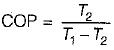T2 = lower temperature
T1 = higher temperature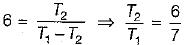QUESTION: 29

A one ton capacity water cooler cools water steadily from 35°C to 20°C. The specific heat of water is 4.18 kJ/kgK. The water flow rate will be nearly

Solution:

Capacity of water cooler = 1 tons = 3.5 kW
T= 35°C
T2 = 20°C
Q = mcΔT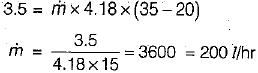QUESTION: 30

In every real refrigeration cycle, ratio of heat absorbed to heat rejected is

Solution: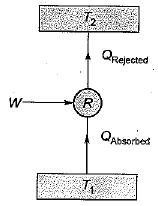Due to work of compression heat rejected is always greater than heat absorbed.
Hence ratio of heat absorbed to heat rejected is less than unity.